## MATH 111 Week 1 Quiz | Assignment Help | University of Washington

MATH 111 Week 1 Quiz | Assignment Help | University of Washington

Question 1

By selecting True:

·         I affirm that my work upholds the highest standards of honesty and academic integrity at the University of Washington and that all answers on this quiz reflect my work and my work alone.

·         I understand that I may reference course materials such as the textbook and my course notes during the quiz.

·         I understand that I am allowed to use a calculator or other calculating device during the quiz.

·         I understand that it is an act of academic misconduct to discuss any of the content of the quiz with another person via any method (text, email, chat).

·         I affirm that have not shared and will not share any information about this quiz with any other person until all parties have submitted their quiz.

o   True

o   False

Question 2

The graph below shows the weight of two Chihuahua puppies for the ten weeks following their birth.

Let A(t) represent the weight of puppy A at time weeks and B(t) represent the weight of puppy at time t weeks.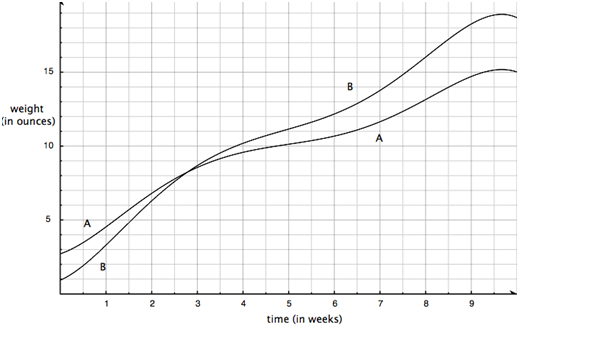Which of the following are equivalent to the incremental rate of change of Puppy A's weight during the h-week period starting at time 7?

Question 3

The graph below shows the weight of two Chihuahua puppies for the ten weeks following their birth.

Let A(t) represent the weight of puppy A at time weeks and B(t) represent the weight of puppy at time t weeks.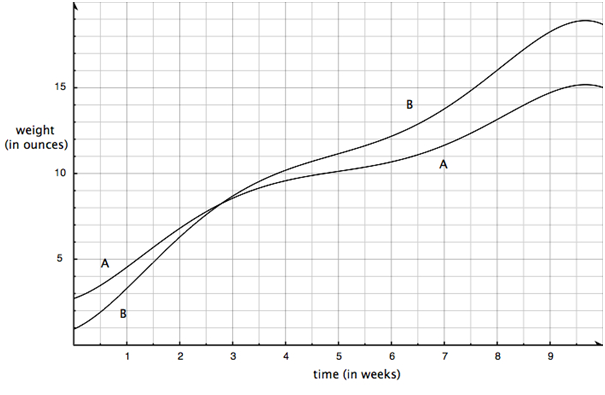Which of the following are equivalent to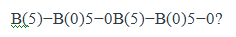Question 4

The graph below shows the weight of two Chihuahua puppies for the ten weeks following their birth.

Let A(t) represent the weight of puppy A at time weeks and B(t) represent the weight of puppy at time t weeks.Which of the following are equivalent to the amount of weight gained by puppy A during the first 6 weeks?

Question 5

Suppose you sell Apples, and suppose that you need to sell 50 Apples in order to maximize profit.

Which of the following are true? (Select all that apply.)

Question 6

Ted sells apples. The graph below shows Ted's total revenue, the line labeled TR.  One of the other lines is Ted's total cost but we don't know which.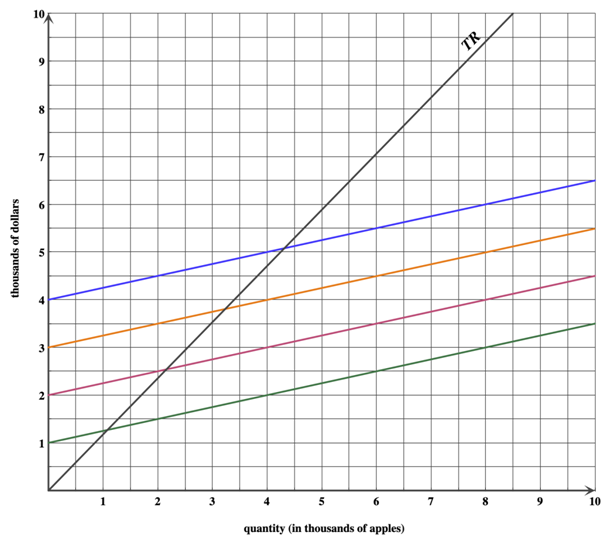Compute the value of marginal cost, MC.MC.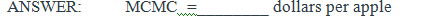Do not include units in the answer blank.  Just a number.  If rounding is necessary, round to the nearest cent.

Question 7

Ted sells apples.  The graph below shows Ted's total revenue, the line labeled TR.  One of the other lines is Ted's total cost.Suppose Ted's fixed costs are 3 thousand dollars.  At what quantity does Ted break even?

ANSWER:  ________ thousand apples

Question 8

Ted sells apples.  The graph below shows Ted's total revenue, the line labeled TR.  One of the other lines is Ted's total cost.Suppose Ted's fixed costs are 2 thousand dollars.  What is Ted's profit if he sells 6000 apples?

ANSWER:  ________ thousand dollars

Question 9

Your company produces Gleblus.  The marginal cost, average cost, and average variable costs for producing Gleblus are shown in the graph below.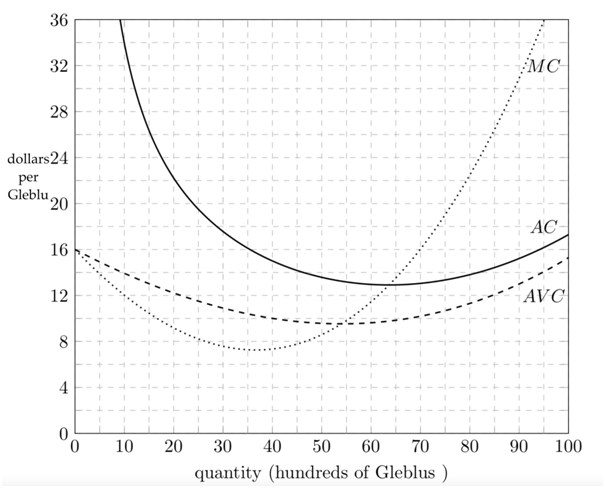Estimate the break even price.

ANSWER:  _________ dollars per Gleblu

Question 10

Your company produces Gleblus.  The marginal cost, average cost, and average variable costs for producing Gleblus are shown in the graph below.Which of the following market prices in dollars for Gleblus should cause you to shut down production? Select all that apply.

Question 11

Your company produces Gleblus.  The marginal cost, average cost, and average variable costs for producing Gleblus are shown in the graph below.Suppose that you sell Glebus at a price of \$24 each. What is the maximum possible profit?

ANSWER:  __________ hundred dollars

### Answer Detail